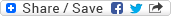## High-Order Methods for Parabolic Equations in Multiple Space Dimensions for Option Pricing Problems

### Dateibereich 6836

14,71 MB in einer Datei, zuletzt geändert am 22.01.2018

DateiDateien geändert amGröße
dc1642.pdf22.01.2018 13:26:2514,71 MB

The pricing of derivatives plays an important role in modern financial markets. The fair value of an option can be computed by solving the corresponding partial differential equation (PDE). In financial engineering these PDEs are in general of convection-diffusion type and are usually multidimensional. Apart from certain exceptions no analytical solution is available and numerical techniques have to be applied.

In this thesis we derive efficient numerical techniques to solve PDEs arising in computational finance. The work is divided into five main parts. In the first section we give a brief introduction to financial option pricing problems. Here, we formulate the PDEs to price European plain vanilla options within the Black-Scholes framework and under stochastic volatility. Further, we briefly discuss various numerical techniques to compute the option price.

In the second section grid based methods to approximate the spatial differential operator are presented. Besides standard and high order compact finite differences, we employ pseudo spectral methods. Since grid based methods suffer from the so called 'curse of dimensionality', we employ the sparse grid combination technique to reduce the number of grid points. The combination technique relies on a certain anisotropic error splitting structure. This motivates our analysis of the splitting structure of finite difference and pseudo spectral schemes. The theoretical investigation of the properties of both approaches is followed by illustrating numerical experiments.

Afterwards we employ Alternating Direction Implicit (ADI) time stepping methods. Four prominent schemes are considered: Douglas, Craig-Sneyd, modified Craig-Sneyd and the Hundsdorfer-Verwer scheme. ADI schemes allow to decompose the spatial discretization matrix into one dimensional problems, which can be solved sequentially. In the case of a high-order compact (HOC) discretization in space we prove bounds for unconditional stability. In the case of pseudo spectral schemes we perform a thorough numerical analysis.

In the fourth section the numerical techniques are applied to PDEs arising in computational finance. We compose different numerical techniques tailored to the regularity of the problem, which makes the schemes especially efficient. In numerical experiments we compare the novel methods to benchmark methods and additionally validate their theoretical properties.

In the last part we summarize the results. Further, we give a concise outlook about possible future research directions.

Lesezeichen:
Permalink |Dokumententyp:
Wissenschaftliche Abschlussarbeiten » Dissertation
Fakultäten und Einrichtungen:
Fakultät für Mathematik und Naturwissenschaften » Mathematik und Informatik » Dissertationen
Dewey Dezimal-Klassifikation:
500 Naturwissenschaften und Mathematik » 510 Mathematik » 510 Mathematik
Sprache:
Englisch
Kollektion / Status:
Dissertationen / Dokument veröffentlicht
Dateien geändert am:
22.01.2018
Datum der Promotion:
31.05.2017
Medientyp:
Text